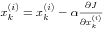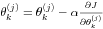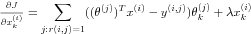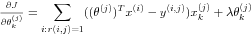# TropicalSoftware Observations

03 April 2020

Posted by PSY

at 1:46 PM

### Recommender Systems

Introduction to Recommender Systems

Recommender systems are an important application of machine learning. They are critical drivers for online retail businesses such as Amazon and Netflix as they introduce customers to products that are purchased by other users with similar tastes. From the perspective of machine learning, it has an important property of learning features.

# When to use Recommender System?

The representative examples are Amazon and Netflix. There are many users, products, ratings and overlap in the items reviewed by different users. So this is not for a one-of-a kind product or a highly customized product for each individual.

The major technique of recommender system is collaborative filtering algorithm. Let's see how collaborative filtering works by applying it to an example of movie ratings.

# Dataset

We use the following matrices to represent a dataset.
nm is the number of movies, nuis the number of users, and n is the number of features.
The matrix Y( a nm x nu matrix) stores the ratings y(i,j) (from 1 to 5, for instance).
The matrix R is an binary-valued indicator matrix, where R(i,j)=1 if user j gave a rating to movie i, and R(i,j)=0 otherwise.
The matrix  ( a nm x n matrix) stores the features of movies. The i-th row of X corresponds to the feature vector x(i) for the i-th movie.
The matrix ( a nu x n matrix) stores user features. The j-th row of Theta corresponds to one parameter vector (j). Both x(i) and (j) are n-dimensional vectors where n is the number of features.

# Objective

We want to predict movie ratings for the movies that users have not yet rated, that is, the entries with R(i,j)=1. This will allow us to recommend the movies with the highest predicted ratings to the user.

# Approach

Consider a set of n-dimensional parameter vectors x(1), ..., xnm and (1), ..., (nu), where the model predicts the rating for movie i by user j as y(i,j) =( (j))Tx(i) (Note: MT means transpose of matrix M). Given a dataset that consists of a set of ratings produced by some users on some movies, the model learns the parameter vectors x(1), ..., xnm and (1), ..., (nu)that produce the best fit (minimizes the squared error).

# Cost Function

By fitting available user ratings, we can estimate parameters such as user features and movie features. The fitting is done by evaluating the cost function given by ,
J(x(1), ..., x(nm), (1), ..., (nu)) = 12(i,j): r(i,j)=1(( (j))Tx(i)-y(i,j))2 + (2j=1nuk=1n(k(j))2 ) + ( 2i=1nmk=1n(xk(j))2 )
The first term on the right measures the squared differences between predictions and available ratings. The second and third terms are for regularization of user parameters and product parameters respectively. Regularization is for minimizing parameters for the sake of generalizing models to fit new data where lambda indicates its strength. So the cost function is determined by a balance between fitting the given data and fitting new data.

The minimization of cost function is done by updating parameters using gradient descent.where is a learning rate which determines the updating speed. The smaller it is, the more precise and slower the updating proceeds.
The gradients of the cost function are calculated by following,# Collaborative filtering learning algorithm

Since we can learn features whether user parameters or product parameters, we can use random numbers for initial parameters and keep updating them until we get the small enough value of cost function. Then we use the learned features to predict a rating. So we can follow these steps for collaborative filtering.
1. Initialize x(1), ..., xnm and (1), ..., (nu)to small random values
2. Minimize J(x(1), ..., x(nm), (1), ..., (nu))using gradient descent for every j=1, ..., nu, i=1, ..., nm
3. For a user with parameters and a movie with learned features x, predict a rating Tx

# Recommendation

For each product i, we learn a feature vector x(i)in Rn.
A feature vector captures the aspects of the product which are important for ratings. For example, the features for movies can be x1 = romance, x2= action, x3= comedy, and so on. If the distance between features of two movies, x(i)-x(j),  is small, we can say the two movies are similar. So We can make recommendations to a user by finding similar items to the one with a high rating by the user.
For a new user who doesn't have any ratings yet, we can predict ratings as the means of available ratings.

# Conclusion

In recommender system using collaborative filtering learning algorithm, each user makes ratings for a subset of products, thus making contributions to learn better features, which are then used to make better predictions of rating for everyone.